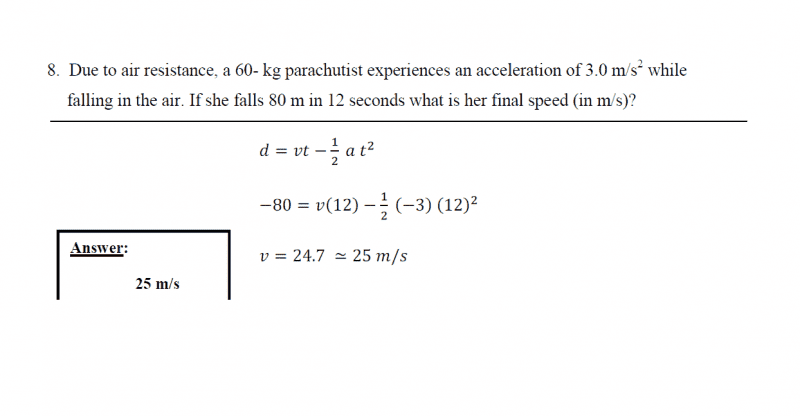# Problem understanding simple constant acceeleration problem

hi

i have an exam and i was trying solving old exams and i came upon this problem

1. Homework Statement

Due to air resistance, a 60- kg parachutist experiences an acceleration of 3.0 m/s2 while
falling in the air. If she falls 80 m in 12 seconds what is her final speed (in m/s)?

## Homework Equations

i know its a constant acceleration problem so
vf=vi+at
D=vit+0.5at^2

## The Attempt at a Solution

D is Y2-Y1 =(-80-0)=-80
by using 2nd equation vi=24.7
and then using 1st equation vf should be -11.3
so final speed should be 11.3
but when i checked the answer i found it different

here is the answer that i found
d=vt-1/2at^2
-80=12v-1/2(-3)(12)^2
v=24.7=25 m/s

Nathanael
Homework Helper
Welcome to Physics Forums.

I'm not sure if your answer is 11.3, or 24.7, but I agree with the answer of 24.7 m/s

Since acceleration is constant, you can find the average speed by taking half of the sum of the initial and final speeds.
In 12 seconds at 3m/s/s, the initial speed would be 36 m/s less than the final speed, so you get $V_{avg}=\frac{1}{2}(V_f+(V_f-36))=V_f-18$
But the average speed is also the total distance divided by the total time so you get $V_{avg}=V_f-18=\frac{80}{12}$
and thus $V_f≈24.7$

Chestermiller
Mentor
Welcome to Physics Forums.

I'm not sure if your answer is 11.3, or 24.7, but I agree with the answer of 24.7 m/s

Since acceleration is constant, you can find the average speed by taking half of the sum of the initial and final speeds.
In 12 seconds at 3m/s/s, the initial speed would be 36 m/s less than the final speed, so you get $V_{avg}=\frac{1}{2}(V_f+(V_f-36))=V_f-18$
But the average speed is also the total distance divided by the total time so you get $V_{avg}=V_f-18=\frac{80}{12}$
and thus $V_f≈24.7$
Don't you think it's a little weird that the initial velocity was upward at 11.3 m/s? Do you think that whoever made up the problem didn't realize this?

Chet

Nathanael
Homework Helper
Don't you think it's a little weird that the initial velocity was upward at 11.3 m/s? Do you think that whoever made up the problem didn't realize this?

Oh wow, I didn't realize this either! Well, that doesn't make much sense...Bystander
Homework Helper
Gold Member
Don't you think it's a little weird
Was looking at it and thought I needed to take a nap. Tried pulling the rip cord at t=0, putting the chute into an updraft. Gave up.

•Nathanael
Chestermiller
Mentor
Was looking at it and thought I needed to take a nap. Tried pulling the rip cord at t=0, putting the chute into an updraft. Gave up.
I think that whoever made up the problem was taking a nap.

Chet

•Nathanael
this was a short problem from last year exam (it's the official answer according to physics department)

so i am not doing something wrong?Chestermiller
Mentor
I can't see how they got this answer. For one thing, the solution to their equation is -24.7, not + 24.7. The equation itself doesn't make sense to me.

Here's how I would have done it (with downward velocities, displacements, and accelerations treated as positive):
$$80 = v_i(12)+\frac{3}{2}(12)^2$$
v_i = -11.33 m/s

v_f = v_i + 3(12) = 24.7 m/s

Nathanael's method in post #2 is equally acceptable (maybe better), and gives the same answer.

Chet

Last edited:
thank you all for clarification